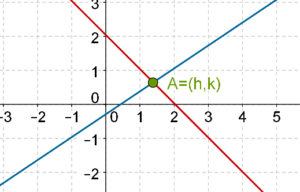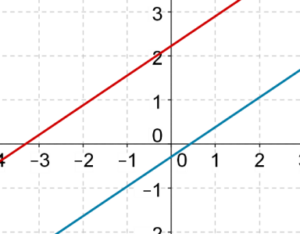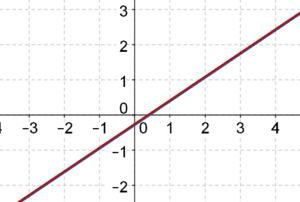# Overview of Graphical Approach in Linear Equations

Overview of Graphical Approach in Linear Equations

Let us summarize the three cases possible with respect to a pair of linear equations.

### Case-1: There is a unique solution

In this case, the two lines will intersect each other at a unique point. The coordinates of that point will be the solution to the pair of linear equations:### Case-2: There is no solution

In this case, the two lines will be parallel to each other, so that there is no common point (of intersection):### Case-3: There are infinitely many solutions

In this case, the two lines happen to coincide. This occurs because the two linear equations are essentially the same, if you remove an appropriate common factor from one of the two equations. In the following figure, we have drawn two lines which coincide, so there appears to be only one line:Pair of Linear Equations in 2 Variables
Pair of Linear Equations in 2 Variables
grade 10 | Questions Set 2
Pair of Linear Equations in 2 Variables
Pair of Linear Equations in 2 Variables
grade 10 | Questions Set 1
More Important Topics
Numbers
Algebra
Geometry
Measurement
Money
Data
Trigonometry
Calculus
More Important Topics
Numbers
Algebra
Geometry
Measurement
Money
Data
Trigonometry
Calculus